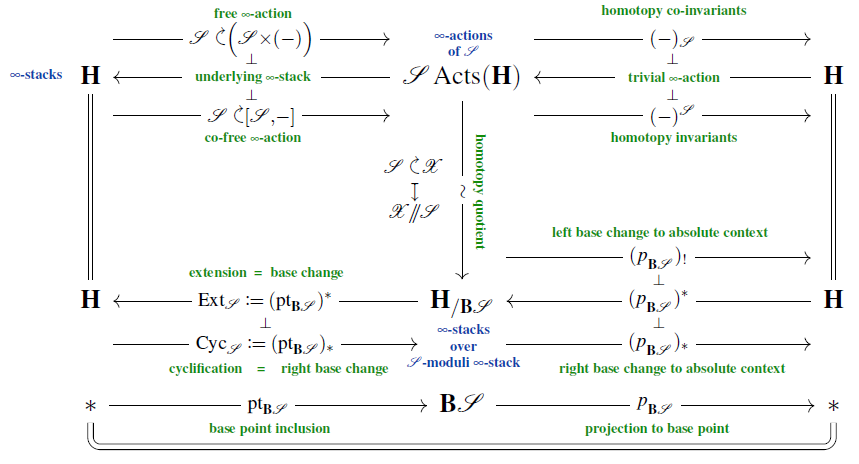Contents

Idea

Any free loop stack $[S^1, \mathcal{X}]$ carries a canonical group action of the circle group $S^1$, given by rigid rotation of loops (explicit models are discussed in Lupercio & Uribe 01, Sec. 3.6). This allows to form the further homotopy quotient/quotient stack by this action:

(1)$Cyc(\mathcal{X}) \;\coloneqq\; [S^1, \mathcal{X}] \!\sslash\! S^1 \,.$

In analogy with cyclic loop spaces, this may be called the cyclic loop stack of $\mathcal{X}$.

Properties

Groupoid presentations

While this is a basic and canonical construction, there seems to be little to no explicit discussion of it in the literature (as of summer 2021). However, the concrete groupoid-presentations considered in Huan 18, Def. 2.5, 2.9, following Ganter 07, Sec. 2.1, may be understood as plausible proposals for models of (1).

Motivated from these definitions is, in turn, that of Huan's inertia orbifolds, whose orbifold K-theory defines a form of equivariant elliptic cohomology of $\mathcal{X}$.

General abstract characterization

Regarding

then (BMSS 19, Sec. 2.2) the cyclic loop stack is equivalently the right base change (dependent product) of $\mathcal{X}$ along the point inclusion $pt_{\mathbf{B}S^1} \colon \ast \to \mathbf{B}S^1$ followed (when desired) by left base change (dependent sum) back to the absolute context:

(2)$Cyc \big( \mathcal{X} \big) \;\; \simeq \;\; \underset{\mathbf{B}S^1 \to \ast}{\sum} \underset{\ast \to \mathbf{B}S^1}{\prod} (\mathcal{X})$From this one may extract component models by invoking the right Quillen adjunction which encodes the derived co-free $\infty$-action, discussed here.

Groupoid models that plausibly represent cyclic loop stacks are discussed (under the notation “$Loops^{ext}$”) in

following